Chapter 15 Electric Forces and Electric Fields Clicker

• Slides: 30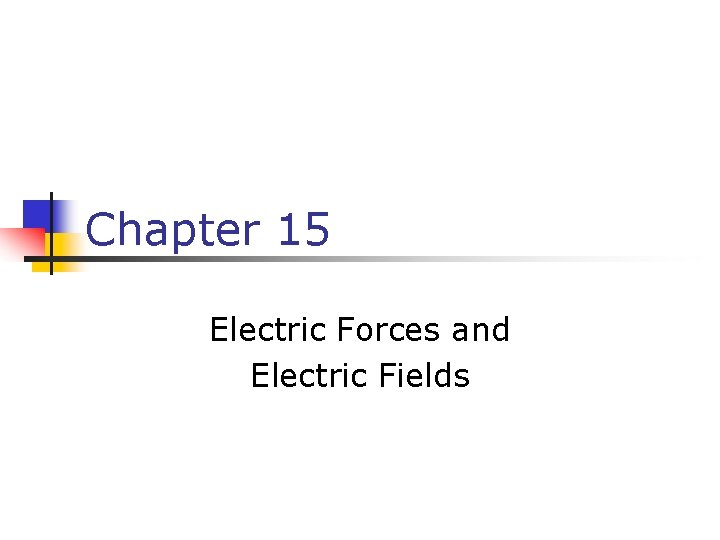Chapter 15 Electric Forces and Electric Fields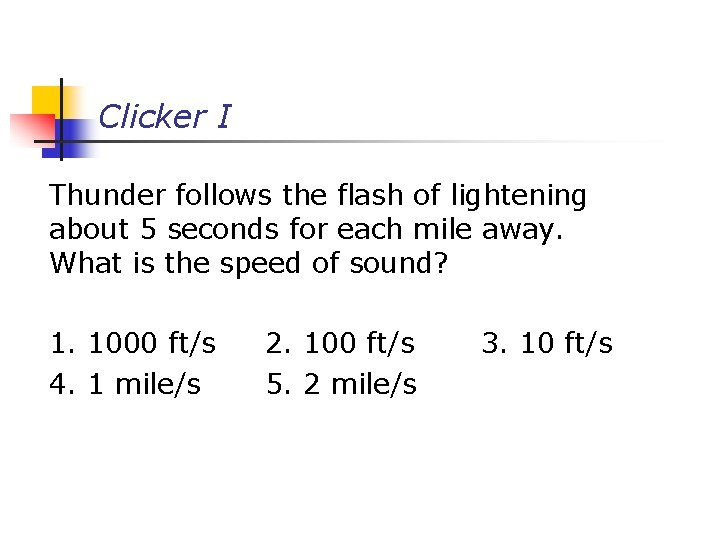Clicker I Thunder follows the flash of lightening about 5 seconds for each mile away. What is the speed of sound? 1. 1000 ft/s 4. 1 mile/s 2. 100 ft/s 5. 2 mile/s 3. 10 ft/s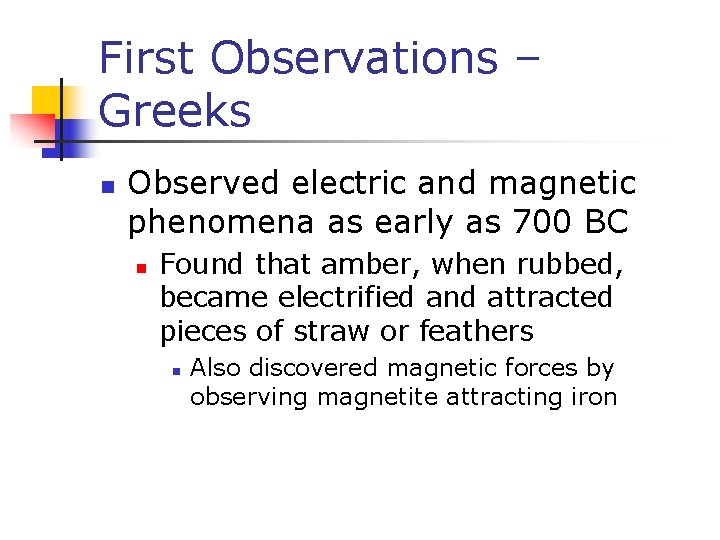First Observations – Greeks n Observed electric and magnetic phenomena as early as 700 BC n Found that amber, when rubbed, became electrified and attracted pieces of straw or feathers n Also discovered magnetic forces by observing magnetite attracting iron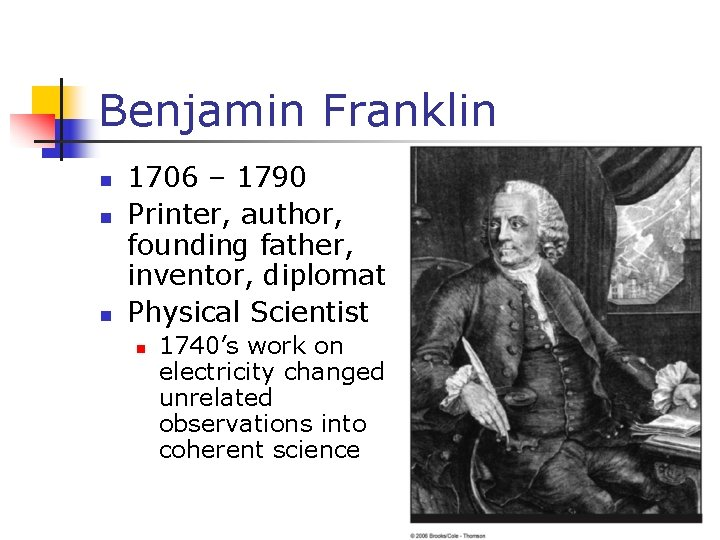Benjamin Franklin n 1706 – 1790 Printer, author, founding father, inventor, diplomat Physical Scientist n 1740’s work on electricity changed unrelated observations into coherent scienceProperties of Electric Charges n Two types of charges exist n n They are called positive and negative Named by Benjamin Franklin Like charges repel and unlike charges attract one another Nature’s basic carrier of positive charge is the proton n Protons do not move from one material to another because they are held firmly in the nucleusMore Properties of Charge n Nature’s basic carrier of negative charge is the electron n n Gaining or losing electrons is how an object becomes charged Electric charge is always conserved n n Charge is not created, only exchanged Objects become charged because negative charge is transferred from one object to another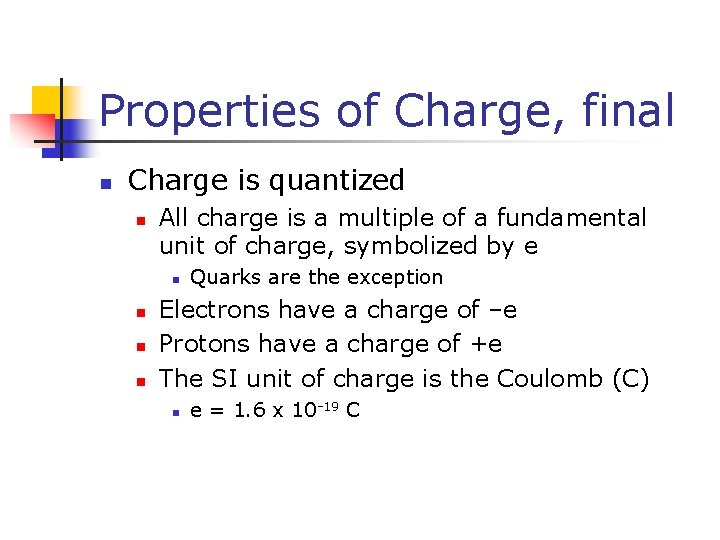Properties of Charge, final n Charge is quantized n All charge is a multiple of a fundamental unit of charge, symbolized by e n n Quarks are the exception Electrons have a charge of –e Protons have a charge of +e The SI unit of charge is the Coulomb (C) n e = 1. 6 x 10 -19 C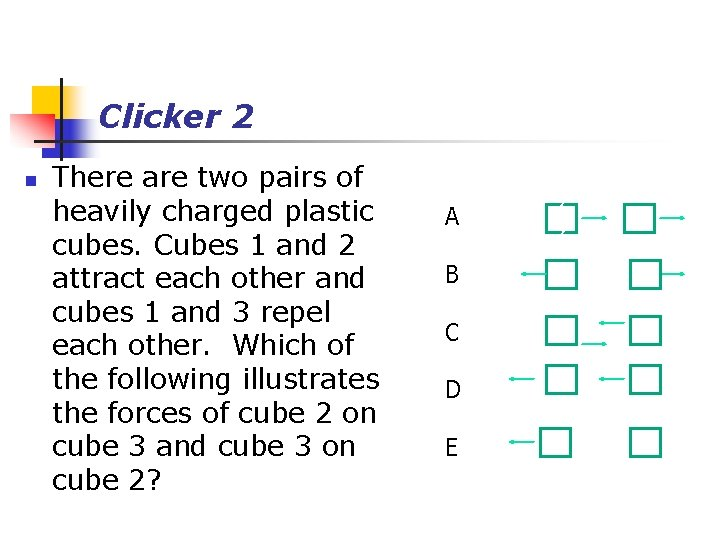Clicker 2 n There are two pairs of heavily charged plastic cubes. Cubes 1 and 2 attract each other and cubes 1 and 3 repel each other. Which of the following illustrates the forces of cube 2 on cube 3 and cube 3 on cube 2? A 2 2 B 2 2 C 2 2 D 2 2 E 2 2Conductors n Conductors are materials in which the electric charges move freely in response to an electric force n n Copper, aluminum and silver are good conductors When a conductor is charged in a small region, the charge readily distributes itself over the entire surface of the materialInsulators n Insulators are materials in which electric charges do not move freely n n Glass and rubber are examples of insulators When insulators are charged by rubbing, only the rubbed area becomes charged n There is no tendency for the charge to move into other regions of the material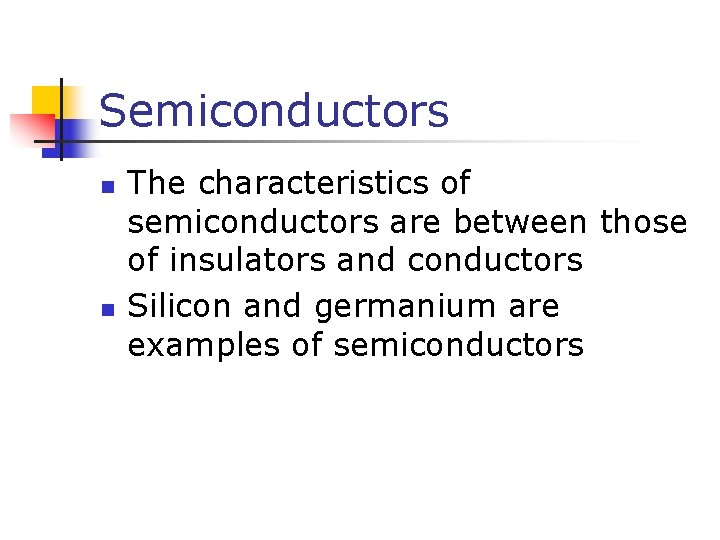Semiconductors n n The characteristics of semiconductors are between those of insulators and conductors Silicon and germanium are examples of semiconductorsCharging by Conduction n n A charged object (the rod) is placed in contact with another object (the sphere) Some electrons on the rod can move to the sphere When the rod is removed, the sphere is left with a charge The object being charged is always left with a charge having the same sign as the object doing the chargingCharging by Induction n n When an object is connected to a conducting wire or pipe buried in the earth, it is said to be grounded A neutral sphere has equal number of electrons and protons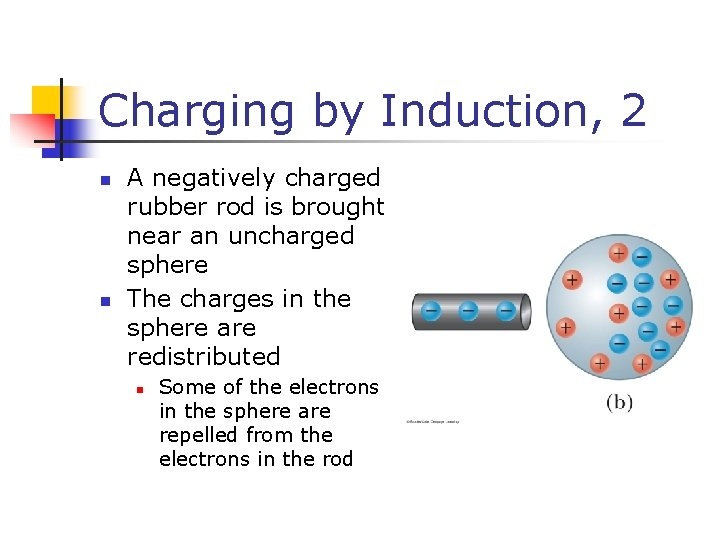Charging by Induction, 2 n n A negatively charged rubber rod is brought near an uncharged sphere The charges in the sphere are redistributed n Some of the electrons in the sphere are repelled from the electrons in the rodCharging by Induction, 3 n n The region of the sphere nearest the negatively charged rod has an excess of positive charge because of the migration of electrons away from this location A grounded conducting wire is connected to the sphere n Allows some of the electrons to move from the sphere to the ground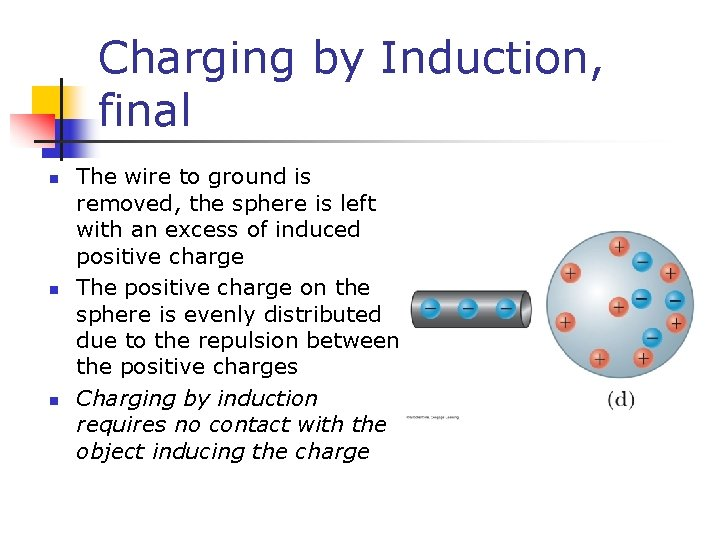Charging by Induction, final n n n The wire to ground is removed, the sphere is left with an excess of induced positive charge The positive charge on the sphere is evenly distributed due to the repulsion between the positive charges Charging by induction requires no contact with the object inducing the chargePolarization n n In most neutral atoms or molecules, the center of positive charge coincides with the center of negative charge In the presence of a charged object, these centers may separate slightly n n This results in more positive charge on one side of the molecule than on the other side This realignment of charge on the surface of an insulator is known as polarization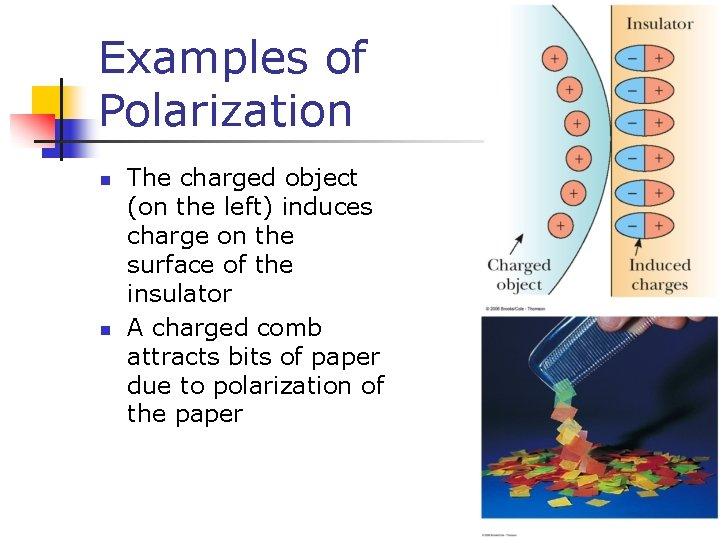Examples of Polarization n n The charged object (on the left) induces charge on the surface of the insulator A charged comb attracts bits of paper due to polarization of the paper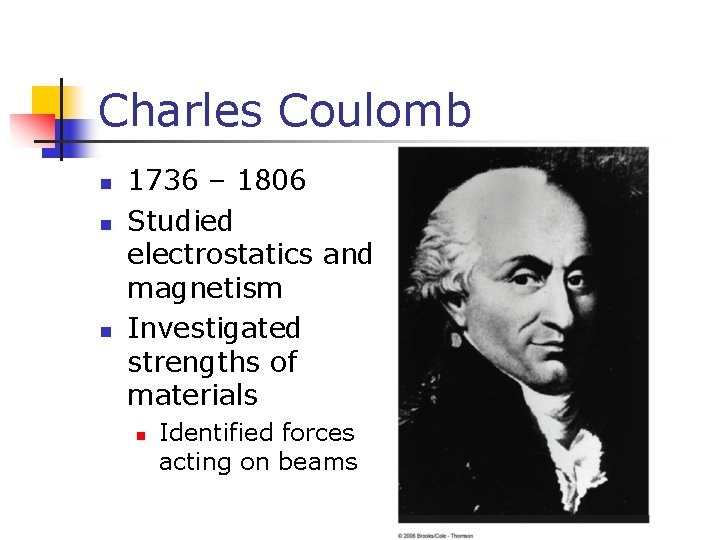Charles Coulomb n n n 1736 – 1806 Studied electrostatics and magnetism Investigated strengths of materials n Identified forces acting on beamsCoulomb’s Law n Coulomb shows that an electrical force has the following properties: n n n It is directed along the line joining the two particles and inversely proportional to the square of the separation distance, r, between them It is proportional to the product of the magnitudes of the charges, |q 1|and |q 2|on the two particles It is attractive if the charges are of opposite signs and repulsive if the charges have the same signsCoulomb’s Law, cont. n Mathematically, n ke is called the Coulomb Constant n n Typical charges can be in the µC range n n n ke = 8. 987 5 x 109 N m 2/C 2 Remember, Coulombs must be used in the equation Remember that force is a vector quantity Applies only to point charges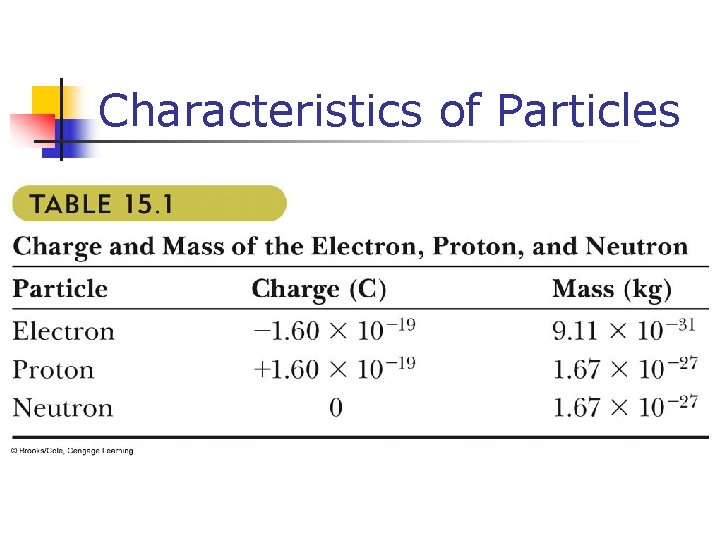Characteristics of Particles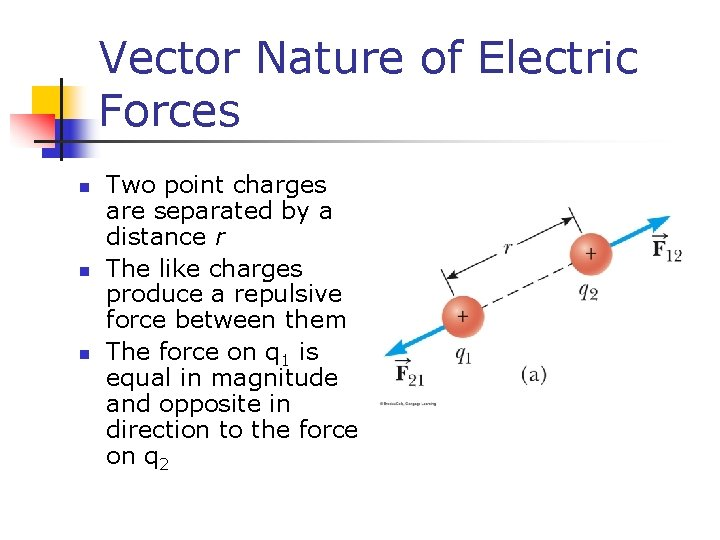Vector Nature of Electric Forces n n n Two point charges are separated by a distance r The like charges produce a repulsive force between them The force on q 1 is equal in magnitude and opposite in direction to the force on q 2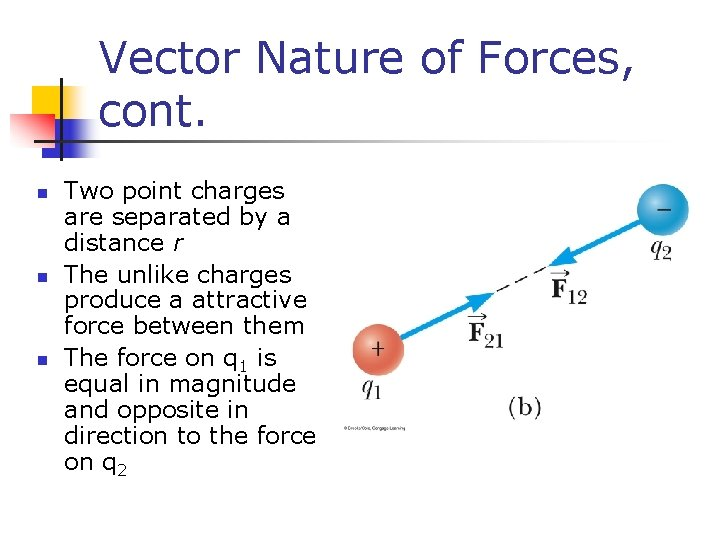Vector Nature of Forces, cont. n n n Two point charges are separated by a distance r The unlike charges produce a attractive force between them The force on q 1 is equal in magnitude and opposite in direction to the force on q 2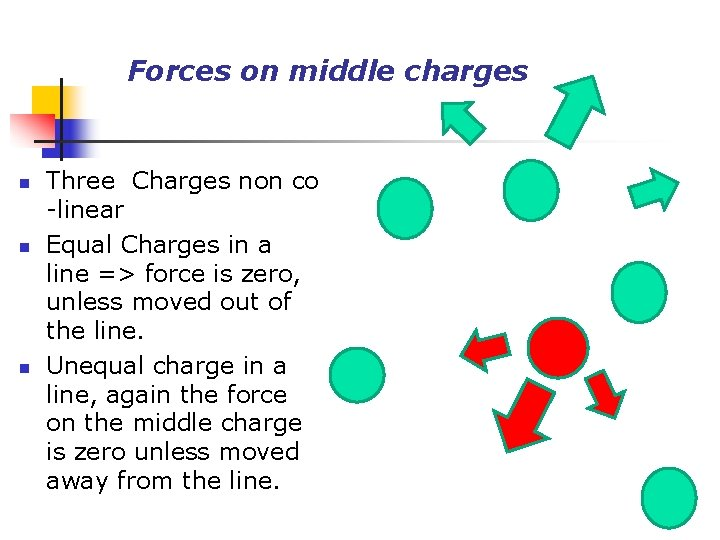Forces on middle charges n n n Three Charges non co -linear Equal Charges in a line => force is zero, unless moved out of the line. Unequal charge in a line, again the force on the middle charge is zero unless moved away from the line.PHY 2054 n n The force on the central charge is zero only if the angle is 30º. Or the charges have different magnitudeElectrical Forces are Field Forces n This is the second example of a field force n n n Gravity was the first Remember, with a field force, the force is exerted by one object on another object even though there is no physical contact between them There are some important similarities and differences between electrical and gravitational forcesElectrical Force Compared to Gravitational Force n n Both are inverse square laws The mathematical form of both laws is the same n n n Masses replaced by charges G replaced by ke Electrical forces can be either attractive or repulsive Gravitational forces are always attractive Electrostatic force is (much) stronger than the gravitational forceThe Superposition Principle n The resultant force on any one charge equals the vector sum of the forces exerted by the other individual charges that are present n n Find the electrical forces between pairs of charges separately Then add the vectors n Remember to add the forces as vectors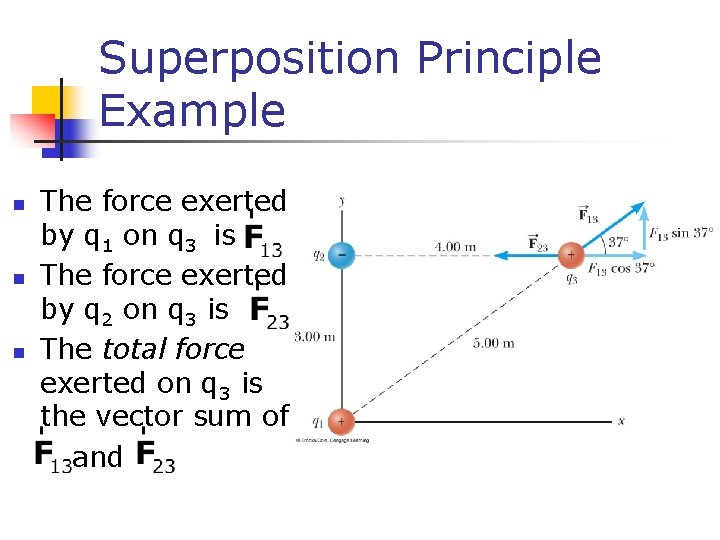Superposition Principle Example n n n The force exerted by q 1 on q 3 is The force exerted by q 2 on q 3 is The total force exerted on q 3 is the vector sum of and